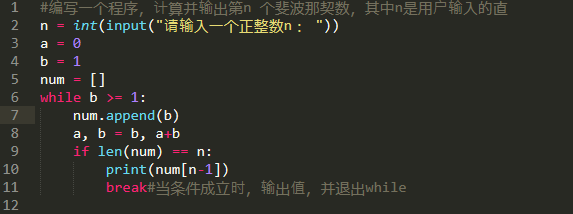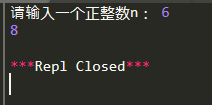• 斐波那契数列又称费波那契数列（意大利语：Successione di Fibonacci），又译为费波拿契数、斐波那契数列、费氏数列、黄金分割数列。在西方，首先研究这个数列的是比萨的列奥那多（意大利人斐波那契Leonardo ...

斐波那契数列又称费波那契数列（意大利语：Successione di Fibonacci），又译为费波拿契数、斐波那契数列、费氏数列、黄金分割数列。在西方，首先研究这个数列的是比萨的列奥那多（意大利人斐波那契Leonardo Fibonacci（1175-1250））,斐波那契在1202年著的《计算之书》中描述了该数列。（百度百科）

一、斐波那契数列定义

在数学上，以递归的形式来定义这个数列。

F0 = 0

F1 = 1

Fn = Fn-1 + Fn-2 (n≥2)

也就是说，该数列的第0项是0，第1项是1，从第2项开始，每项都是前两项的和。

很多教科书中在描述该问题时都是从第1项开始的，也就是说：

F1 = 1

F2 = 1

Fn = Fn-1 + Fn-2 (n≥3)

二、该问题在Python中的实现

下面给出再Python中的实现方法：

1、列表版

N = 40 #输出项数

fn = [1,1] #定义前两项

i = 2 #从第3项开始计数# 循环赋值

while

i < N:

fn.append(fn[ i - 1 ] + fn[ i - 2 ]) # 每项是前两项的和

i += 1 #循环变量递增

for

e

in

fn: # 迭代输出列表中的每一项

print(e)

输出结果（结果较长，这里省略了中间的项）：

1

1

2

3

5

8

13

21

...

24157817

39088169

63245986

1023341552、变量版

f1 = 1 # 第1项

f2 = 1 # 第2项

i = 2 # 从第3项开始循环

N = 40 / 2 #因为每次输出两项，所以除以2

# 每次输出两项，因为在循环开始时输出

# 会造成最后两项不会输出，所以这里要+1

while

i <= N + 1:

print(f1,",",f2)

f1 = f1 + f2 # 第n项

f2 = f1 + f2

# 第n + 1项

i += 1 # 循环计数+1

输出结果：

1 , 1

2 , 3

5 , 8

13 , 21

34 , 55

89 , 144

233 , 377

610 , 987

1597 , 2584

4181 , 6765

10946 , 17711

28657 , 46368

75025 , 121393

196418 , 317811

514229 , 832040

1346269 , 2178309

3524578 , 5702887

9227465 , 14930352

24157817 , 39088169

63245986 , 1023341553、简洁版

f1,f2 = 0, 1

N = 40

i = 2

while

i <= N + 1:

print (f2, end = ' ')

f1,f2 = f2, f1 + f2

这个算法会把所有的项输出到一行中，每个用空格隔开。

输出结果：

1 1 2 3 5 8 13 21 34 55 89 144 233 377 610 987 1597 2584 4181 6765 10946 17711 28657 46368 75025 121393 196418 317811 514229 832040 1346269 2178309 3524578 5702887 9227465 14930352 24157817 39088169 63245986 102334155

4、控制每行输出的数量

f1,f2 = 0,1

N = 40

i = 2

j = 0

num_per_row = 5

while

i <= N + 1:

print(f2,end = ' ')

j += 1

if

j % num_per_row == 0:

print('\n',end = '')

f1,f2 = f2, f1 + f2

i += 1

输出结果：

1 1 2 3 5

8 13 21 34 55

89 144 233 377 610

987 1597 2584 4181 6765

10946 17711 28657 46368 75025

121393 196418 317811 514229 832040

1346269 2178309 3524578 5702887 9227465

14930352 24157817 39088169 63245986 102334155

5、使用函数输出第n项

def

Fibonacci(n):

if

n < 0:

raise

IndexError('参数不能小于0。')

if

n == 0:

return

0

elif

n <= 2:

return

1

else:

return

Fibonacci(n - 1) + Fibonacci(n - 2)

v = Fibonacci(30)

print(v)

输出结果：

832040以上介绍了Python中计算Fibonacci数列的相关算法，如有不妥之处，请留言评论。如需转载，请注明出处和本文链接：翔宇亭IT乐园（http://www.biye5u.com/）

本文链接地址：http://www.biye5u.com/article/python/2020/6470.html

展开全文• 运行结果：运行结果：展开全文Python
• #define _CRT_SECURE_NO_WARNINGS 1 #include<...int fun1(int n) //函数计算斐波那契数列 { if (n <= 2) return 1; else return fun1(n - 1) + fun1(n - 2); } int fun(int n) { int a =...

#define _CRT_SECURE_NO_WARNINGS 1

#include<malloc.h>  //动态内存分配.h文件

#include<stdio.h>

int fun1(int n) //函数 计算斐波那契数列
{
if (n <= 2)

return 1;
else
return fun1(n - 1) + fun1(n - 2);

}
int fun(int n)
{
int a = 1;
int b= 1;
int c= 1;
while (n > 2) //while循环，计算斐波那契，可省去递归的多余计算
{
c = a + b;
a = b;
b = c;
n--;

}
return c;

}

int main()  //函数返回计算斐波那契
{
int n = 0;
scanf("%d", &n);
printf("n is:%d\n", fun(n));
return 0;
}

展开全文c# c++ c语言 java python
• 问题描述：斐波那契数列是这样一个数列：1、1、2、3、5、8、13、21…第n个数是第（n-1）个数和第（n-2）个数的和； f=[1 1]; k=1; while f(k)<10000 f(k+2)=f(k)+f(k+1); k=k+1; end k f(k) k = 21 ans = ...

问题描述：斐波那契数列是这样一个数列：1、1、2、3、5、8、13、21…第n个数是第（n-1）个数和第（n-2）个数的和；

f=[1 1];
k=1;
while f(k)<10000
f(k+2)=f(k)+f(k+1);
k=k+1;
end
k
f(k)
k =
21
ans =
10946
展开全文• var i = 1; var j = 1; var sum = i + j; while (i < 100) { var s = i + j; i = j; j = s; sum += s; } console.log(sum);
• <!DOCTYPE html> <title></title> document.write("第一题：输出前20项斐波拉契数：");... var a=1,b=1,c;... while(i); 转载于:https://www.cnblogs.com/yan-linxi/p/8057126.html
•斐波那契 Java 数组
• 今天利用三种不同循环语句来编写100以内的斐波那契数列 首先利用for循环语句 #include int main() { int a={1,2}; int i; printf("%d",a); for(i=2;a[i-1];i++) { } }c语言
• n1 = 1 n2 = 1 n3 = 1 if n ('输入有误') return -1 while (n - 2) > 0: n3 = n2 + n1 n1 = n2 n2 = n3 n = n - 1 return n3 def main(): res = f(98) print(res) if __name__ == '__main__': main() result /home/...
• 斐波那契数列（Fibonacci sequence）是1、1、2、3、5、8、13、21、34、55... 对于斐波那契数列的第n项求解，通常是使用递归方式实现，如下 public static int fbNum(int n) { if (n==1 || n==2) return 1; ...动态规划 leetcode java 数据结构
• Python3基础 用 while循环实现 斐波那契数列 镇场诗: 诚听如来语,顿舍世间名与利.愿做地藏徒,广演是经阎浮提. 愿尽吾所学,成就一良心博客.愿诸后来人,重现智慧清净体.-------------------------------------- ... ...
• C语言数据结构递归之斐波那契数列因为自己对递归还是不太熟练，于是做POJ1753的时候就很吃力，就是翻棋子直到棋盘上所有棋子的颜色一样为止，求最少翻多少次，方法是枚举递归。然后就打算先做另一道递归的题(从数组...
• 定义：斐波那契数列指的是这样一个数列：0, 1, 1, 2, 3, 5, ...以输出斐波那契数列的前20项为例：方法一：比较标准的做法，是借助第三个变量实现的。#include using namespace std;int main(){ int f1=0,f2=1,t,n=1;...
• ：def fibonacci(n, a=0, b=1):if a >= n : return [a]return [a] + fibonacci(n,b,a+b)[编辑]以下是它的工作原理：该函数通过向下一次调用自身的结果添加一个元素[a]来逐步构建数组。第一行允许它在达到目标时...
• 斐波那契数列，简单地说，起始两项为0和1，此后的项分别为它...) 实现方式一：根据这一特性，可采用最简单的方法计算该项，循环计算每项的值，以下为python实现代码，输出该数列的前num项# -*- coding:utf-8 -*-def ...
• 用matlab实现fibonacci数列，比较简单，对matlab的初学者非常有用，
• while循环 while ( 循环条件 ) { 循环操作 } do while循环 do { 循环操作 } while ( 循环条件 ); 特点：先执行，再判断 while循环和do-while循环的区别 执行次序不同 初始情况不满足循环条件时 while循环一次都...
• 概述今天主要分享下怎么用Python实现斐波那契数列，大家有空可以玩一下~需求用Python脚本实现斐波那契数列。...如果图方便就递归实现，图性能就用循环。非递归实现打印斐波那契数列#usr/bin/python## 斐波那契数...
• 递归效率低，除了最后一个数，每个数都被重复计算若干次1: //递归实现2: public static int Fib1(int n)3: {4: if (n < 3)5: {6: return 1;7: }8: else9: {10: return Fib1(n - 1) + Fib1(n - 2);...
•编程语言 c语言基础 教学 光天化日学C语言 while 循环
• for和while循环

2021-07-15 20:53:36
循环 循环 - 让代码重复执行(代码写一遍,运行的时候可以执行多次) print('hello') print('hello') print('hello') print('hello') print('hello') 一、 for循环 语法： for 变量 in 序列： 循环体(需要重复执行的...python
• b, c, d） 功能： 从左到右执行 最终表达式的值是最后一项的值 while 1，执行顺序 格式： while（表达式）{ 语句 } 2，与for的相互比较 for和while可以相互转化 for的逻辑性更强，更不容易出错，推荐使用for循环 3...
• 菲波那切数列指的是这样一个数列：1、1、2、3、5、8、13、21、34、…… 在数学上，斐波纳契数列以如下被以递归的方法定义：F(1)=1，F(2)=1, F...int Fibonacci_1(int N) { if(N == 1 || N == 2) return 1; else...数据结构 菲波那切数列 递归 迭代
• 第三步：利用for循环打印第三位及以后的斐波那契数，这里不用建议while，因为循环的次数可以通过键盘输入确定 循环条件中，i初始化的值为3，是因为从第三位开始输出斐波那契数，这样才会保证输java
• while循环语法如下：while(布尔表达式){执行语句;//也可以是语句块}先判断表达式是否为true，只要布尔表达式为 true，循环就会一直执行下去，反之，跳出循环。看下面例子：从上面的例子可以看出，我们可以设置一个...
• R语言循环语句1、for语句2、while循环语句3、repeat语句4、举例5、引用 1、for语句 R语言批量处理1——输出list文件及数据框长宽转换 R语言批量处理2——导入excel，定位提取合并为数据框data.frame 2、while循环...r语言 for循环 循环语句
• 斐波那契数列的前四十项（不用数组实现） #include <stdio.h> #include <stdlib.h> static void test(void) { long int f1 = 1,f2 = 1; int i; for(i = 1;i <= 20;i++) { printf("%12d %12d",...c语言 linux
• while循环 for循环 语法： for 变量 in 序列： ​ 循环体 说明 for in 关键字 固定写法 变量 变量名（可以是定义过得变量，一般是没有定义过的变量） 序列 容器型数据类型得数据 （字符串、列表 字典 元组 迭代器 ...python...